## Tuesday, May 26

### Facebook Emoticons / Emoji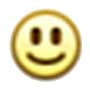` :)`` :D`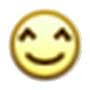` ^_^`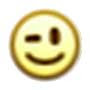` ;)`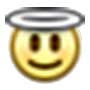` O:)`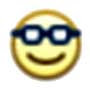` 8-)`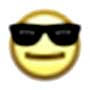` 8|`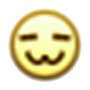` :3`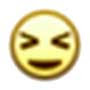` >:O`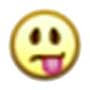` :P`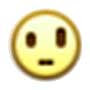` o.O`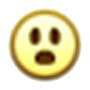` :O`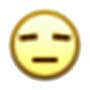` -_-`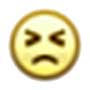` >:(`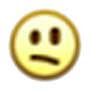` :/`` :'(`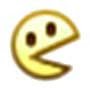`:v`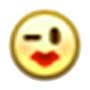` :*`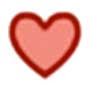` <3`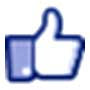` (y)`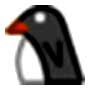` <(")`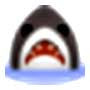` (^^^)`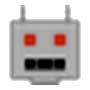` :|]`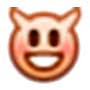` 3:)`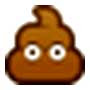` :poop:`